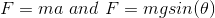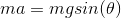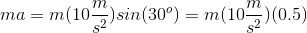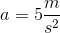# AP Physics B : Understanding Accelerations

## Example Questions

### Example Question #2 : Ap Physics 1

If a 15kg object is subjected to a force of 175N to the east and another force of 1020N to the west, what is the object’s acceleration?

5.6m/s2

56m/s2

1.4m/s2

113m/s2

56m/s2

Explanation:

First, find the net force by subtracting the opposing forces.

F = 1020N – 175N = 845 N to the west

Next, find acceleration using Newton's second law,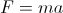.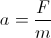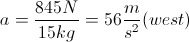### Example Question #3 : Displacement, Velocity, And Acceleration

Three blocks (in left-to-right order: block A, block B, block C) are pushed along a frictionless horizontal surface by a 50N force to the right, which acts on block A.

If the masses of the blocks are given by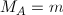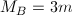, and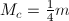, which of the following best describes the relationship between the accelerations of the blocks?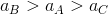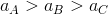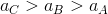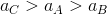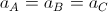Explanation:

The three blocks must remain in contact as they move, so they will each have the same velocity and acceleration regardless of their different masses. So,.

### Example Question #1 : Motion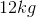block slides down a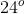incline. If the coefficient of friction between the block and the incline is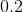, determine the acceleration of the block as it slides down the incline.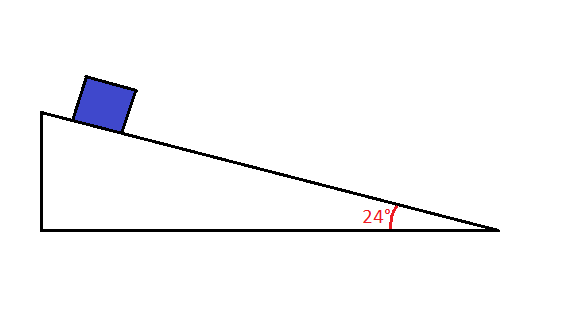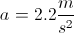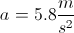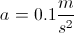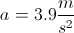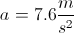Explanation:

Know what forces are involved by drawing a force diagram.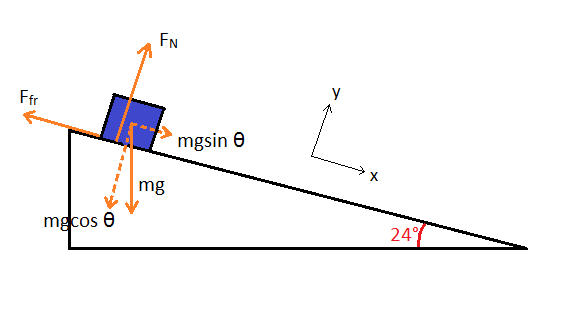The gravitational force is broken into the x and y components. The net force on the block in the y-direction is the normal force minus the y component of the gravitational acceleration (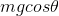).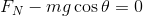Notice that the net y-force is equal to zero to show that the block is not moving anywhere in the y-direction. Now, we can isolate the normal force.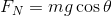The net force in the x-direction is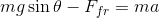. We know that the block is accelerating in the x-direction; therefore the net force is equal to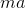.

We can use the friction equations to substitute for the x-direction forces.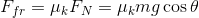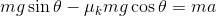We can isolate the acceleration and solve using the provided values.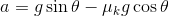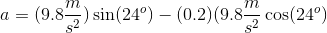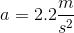### Example Question #1 : Newtonian Mechanics

A car of mass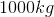is initially at rest, and then accelerates at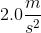for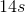. What is the kinetic energy of the car at time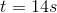?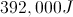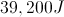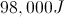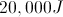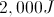Explanation:

The first step will be to find the final velocity of the car. We know the acceleration and time, so we can find the final velocity using kinematics. The initial velocity is zero, since the car starts at rest.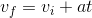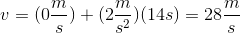Use this velocity and the mass of the car to solve for the final kinetic energy.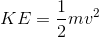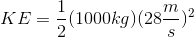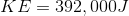### Example Question #1 : Motion

A man throws a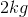ball straight up in the air at a velocity of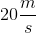. If there is a constant air resistance force of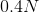against the motion of the ball, what is the maximum height of the ball?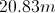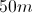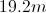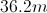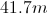Explanation:

We first need to find the net force acting on the ball during flight. We can then use the net force and Newton's second law to find the total acceleration on the ball.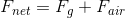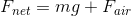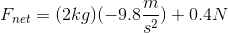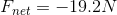Use this net force to find the acceleration.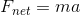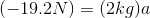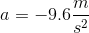From here, there are two ways to solve. One way uses kinematic equations, and the other uses energy. We will solve using energy.

Total energy must be conserved during the throw, so the initial kinetic energy must equal the final potential energy (since velocity is zero at the maximum height).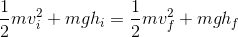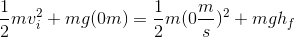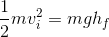Use the given initial velocity to find the final height.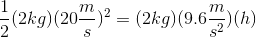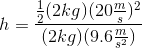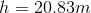### Example Question #6 : Ap Physics 1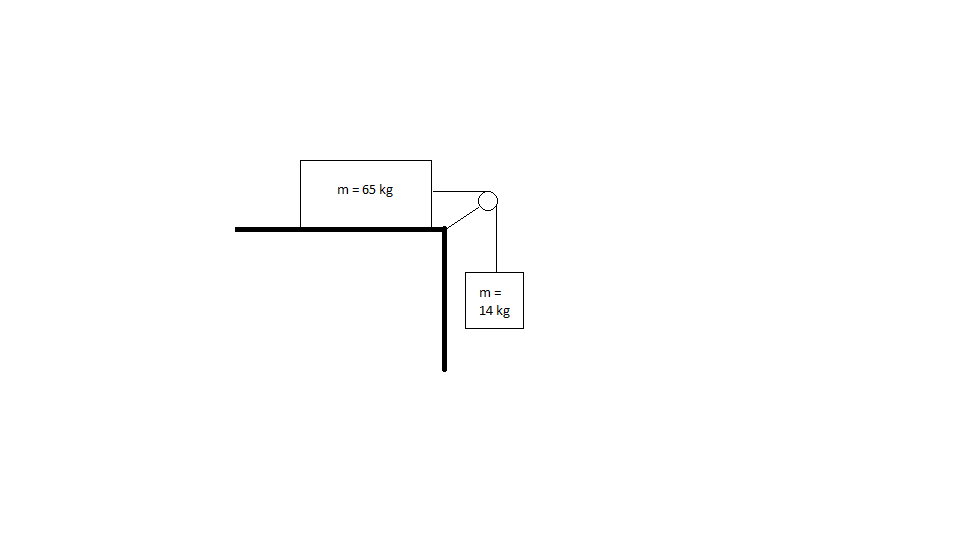What is the acceleration of the system shown above? (Assume the table is frictionless and the mass of the rope connecting blocks is negligible).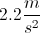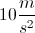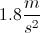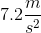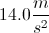Explanation:

The force that translates to the entire system is that of gravity acting on the mass hanging over the ledge.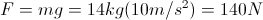140N is the total force acting on the system, which has a mass equal to both blocks combined (65 kg + 14 kg = 79 kg). We can find the acceleration using Newton's second law.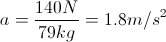### Example Question #2 : Linear Motion

A car moving at 40m/s suddenly applies a braking force and comes to rest in 20s, with constant deceleration. If the car has a mass of 2000kg, what is the braking force?

–2000N

–8000N

4000N

40000N

–4000N

–4000N

Explanation:

First we can calculate the acceleration.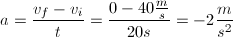Using F = ma with the magnitude of the acceleration we can find the force.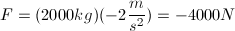### Example Question #3 : Linear Motion

A box is placed on a 30o frictionless incline. What is the acceleration of the box as it slides down the incline?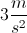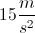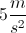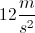Explanation:

To find the acceleration of the box traveling down the incline, the mass is not needed. Using the incline of the plane as the x-direction, we can see that there is no movement in the y-direction; therefore, we can use Newton's second, F = ma, in the x-direction.

There is only one force in the x-direction (gravity), however gravity is not just equal to “mg” in this case. Since the box is on an incline, the gravitational force will be equal to mgsin(30o). Substituting force into F  =ma we find that mgsin(30o) = ma. We can now cancel out masses and solve for acceleration.